# Samacheer Kalvi Books: Tamilnadu State Board Text Books Solutions

## Samacheer Kalvi 11th Chemistry Chapter 4 Hydrogen Notes PDF Download: Tamil Nadu STD 11th Chemistry Chapter 4 Hydrogen NotesSamacheer Kalvi 11th Chemistry Chapter 4 Hydrogen Notes PDF Download: Tamil Nadu STD 11th Chemistry Chapter 4 Hydrogen Notes

We bring to you specially curated Samacheer Kalvi 11th Chemistry Chapter 4 Hydrogen Notes PDF which have been prepared by our subject experts after carefully following the trend of the exam in the last few years. The notes will not only serve for revision purposes, but also will have several cuts and easy methods to go about a difficult problem.

 Board Tamilnadu Board Study Material Notes Class Samacheer Kalvi 11th Chemistry Subject 11th Chemistry Chapter Chapter 4 Hydrogen Format PDF Provider Samacheer Kalvi Books

## How to Download Samacheer Kalvi 11th Chemistry Chapter 4 Hydrogen Notes PDFs?

2. Click on the Samacheer Kalvi 11th Chemistry Notes PDF.
3. Look for your preferred subject.
4. Now download the Samacheer Kalvi 11th Chemistry Chapter 4 Hydrogen notes PDF.

## Download Samacheer Kalvi 11th Chemistry Chapter 4 Hydrogen Chapterwise Notes PDF

11th Chemistry Chapter 4 Book Back Answers Question 1.
Which of the following statements about hydrogen is incorrect ? (NEET – 2016)
(a) Hydrogen ion, H3O+ exists freely in solution.
(b) Dihydrogen acts a,s a reducing agent.
(c) Hydrogen has three isotopes of which tritium is the most common.
(d) Hydrogen never acts as cation in ionic salts.
(c) Hydrogen has three isotopes of which tritium is the most common.
Hint:
Correct statement:
Hydrogen has three isotopes of which protium is the most common.

11th Chemistry Lesson 4 Book Back Answers Question 2.
Water gas is ………..
(a) HO(g)
(b) CO + H2O
(C) CO + H2
(d) CO + N2
(c) CO + H2

11th Chemistry 4th Lesson Book Back Answers Question 3.
Which one of the following statements is incorrect with regard to ortho and para dihydrogen ?
(a) They are nuclear spin isomers
(b) Ortho isomer has zero nuclear spin whereas the para isomer has one nuclear spin
(c) The para isomer is favoured at low temperatures
(d) The thermal conductivity of the para isomer is 50% greater than that of the ortho isomer.
(b) Ortho isomer has zero nuclear spin whereas the para isomer has one nuclear spin
Hints:
Correct statement:
Ortho isomer – one nuclear spin Para isomer – zero nuclear spin

11th Chemistry Unit 4 Book Back Answers Question 4.
Ionic hydrides are formed by …………….
(a) halogens
(b) chalogens
(c) inert gases
(d) group one elements
(d) group one elements
e.g., Sodium hydride (Na+ H )

Chemistry Class 11 Samacheer Kalvi Question 5.
Tritium nucleus contains ……………..
(a) 1p + 0n
(b) 2p + 1n
(c) 1p + 2n
(d) none of these
(c) lp + 2n
1T3 (le, lp, 2n)

Class 11 Chemistry Solutions Samacheer Kalvi Question 6.
Non-stoichiometric hydrides are formed by……………..
(b) carbon, nickel
(c) manganese, lithium
(d) nitrogen, chlorine

11th Chemistry Hydrogen Lesson Question 7.
Assertion : Permanent hardness of water is removed by treatment with washing soda.
Reason : Washing soda reacts with soluble calcium and magnesium chlorides and sulphates in hard water to form insoluble carbonates
(a) Both assertion and reason are true and reason is the correct explanation of assertion.
(b) Both assertion and reason are true but reason is not the correct explanation of assertion.
(c) Assertion is true but reason is false
(d) Both assertion and reason are false
(a) Both assertion and reason are true and reason is the correct explanation of assertion.
Ca2+ + Na2CO3 → CaCO3↓ + 2Na+

11th Chemistry 4th Chapter Question 8.
If a body of a fish contains 1.2 g hydrogen in its total body mass, if all the hydrogen is replaced with deuterium then the increase in body weight of the fish will be ……………
(a) 1.2 g
(b) 2.4 g
(c) 3.6 g
(d) 4.8−−−√ g
(a) 1.2 g
Hints:
Mass of deuterium = 2 × mass of protium
If all the 1.2 g hydrogen is replaced with deuterium, the weight will become 2.4g. Hence the increase in body weight is (2.4 – 1.2 = 1.2 g).

Samacheer Kalvi 11th Chemistry Book Solutions Question 9.
The hardness of water can be determined by volumetrically using the reagent …………..
(a) sodium thio sulphate
(b) potassium permanganate
(c) hydrogen peroxide
(d) EDTA
(d) EDTA

Hydrogen 11th Chemistry Question 10.
The cause of permanent hardness of water is due to ………….
(a) Ca(HCO3)2
(b) Mg(HCO3k)3
(c) CaCl2
(d) MgCO3
(c) CaCl2
Hints:
Permanent hardness if water is due to the presence of the chlorides, nitrates and sulphates of Ca2+ and Mg2+ ions.

Hydrogen Class 11 Questions And Answers Question 11.
Zeolite used to soften hardness of water is, hydrated ………….
(a) Sodium aluminium silicate
(b) Calcium aluminium silicate
(c) Zinc aluminium borate
(d) Lithium aluminium hydride
(a) Sodium aluminium silicate
Zeolite is sodium aluminium silicate.
(NaAlSi2O6 .H2O)

11th Chemistry Deleted Book Back Questions Question 12.
A commercial sample of hydrogen peroxide marked as 100 volume H2O2, it means that ……………
(a) 1 ml of H2O2 will give 100 ml O2 at STP
(b) 1 L of H2O2 will give 100 ml O2 at STP
(c) 1 L of H2O2 will give 22.4 L O2
(d) 1 ml of H2O2 will give 1 mole of O2 at STP
(a) 1 ml of H2O2 will give 100 ml O2 at STP

Samacheer Kalvi Guru 11th Chemistry Question 13.
When hydrogen peroxide is shaken with an acidified solution of potassium dichromate in presence of ether, the ethereal layer turns blue due to the formation of
(a) Cr2 O3
(b) CrO42-
(c) CrO(O2)2
(d) none of these
(c) CrO(O2)2 CrO(O2)2
Hints:
Cr2O72- + 2H+ + 4H2O2 → 2CrO(O2)2 + 5H2O

Samacheer Kalvi 11th Chemistry Question 14.
For de colorization of 1 mole of acidified KMnO4, the moles of H2O2 required is …………….
(a) 12
(b) 32
(c) 52
(d) 72
(c) 52
Hints:
2MnO4 + 5H2O2(aq) + 6H+ → 2Mn2++ 5O2 + 8H2O

11th Chemistry Samacheer Kalvi Question 15.
Volume strength of 1.5 N H2O2 is ……………..
(a) 1.5
(b) 4.5
(c) 16.8
(d) 8.4
(d) 8.4
Hints:
Volume strength of hydrogen peroxide = Normality of hydrogen peroxide × 5.6 = 1.5 x 5.6 = 8.4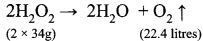Volume strength of hydrogen peroxide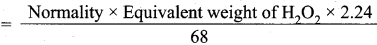= Normality × 17×22.468
Volume strength of hydrogen peroxide = Normality x 5.6

Samacheer Kalvi Chemistry 11th Question 16.
The hybridization of oxygen atom is H2O and H2O2 are respectively
(a) sp and sp3
(b) sp and sp
(c) sp and sp2
(d) sp3 and sp3
(d) sp3 and sp3

Samacheer Kalvi 11th Chemistry Solutions Question 17.
The reaction H3PO2 + D2O → H2DPO2 + HDO indicates that hypo-phosphorus acid is ……………
(a) tri basic acid
(b) di basic acid
(c) mono basic acid
(d) none of these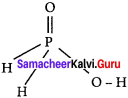(c) mono basic acid
Hints:
Hypophosphorus acid on reaction with D2O, only one hydrogen is replaced P by deuterium and hence it is mono basic.

Samacheer Kalvi Class 11 Chemistry Solutions Question 18.
In solid ice, oxygen atom is surrounded
(a) tetrahedrally by 4 hydrogen atoms
(b) octahedrally by 2 oxygen and 4 hydrogen atoms
(c) tetrahedrally by 2 hydrogen and 2 oxygen atoms
(d) octahedrally by 6 hydrogen atoms
(a) tetrahedrally by 4 hydrogen atoms

Samacheer Kalvi 11th Chemistry Guide Pdf Question 19.
The type of H-bonding present in ortho nitro phenol and p-nitro phenol are respectively ……………
(a) inter molecular H-bonding and intra molecular H-bonding
(b) intra molecular H-bonding and inter molecular H-bonding
(c) intra molecular H – bonding and no H – bonding
(d) intra molecular H – bonding and intra molecular H – bonding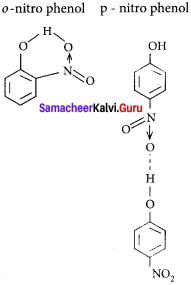(A) intra molecular H-bonding and inter molecular H-bonding

11 Chemistry Samacheer Kalvi Question 20.
Heavy water is used as ……………
(a) modulator in nuclear reactions
(b) coolant in nuclear reactions
(c) both (a) and (b)
(d) none of these
(c) both (a) and (A)
Hints:
Heavy water is used as moderator as well as coolant in nuclear reactions.

Samacheer Kalvi.Guru 11th Chemistry Question 21.
Water is a ……………
(a) basic oxide
(b) acidic oxide
(c) amphoteric oxide
(d) none of these
(c) amphoteric oxide

II. Write brief answer to the following questions

Question 22.
Explain why hydrogen is not placed with the halogen in the periodic table.

• Hydrogen resembles alkali metals as well as halogens.
• Hydrogen resembles more alkali metals than halogens.
• Electron affinity of hydrogen is much less than that of halogen atom. Hence the tendency to form hydride ion is low compared to that of halogens.
• In most of its compounds hydrogen exists in +1 oxidation state. Therefore it is reasonable to place the hydrogen in group 1 along with alkali metals as shown in the latest periodic table published by IUPAC.

Question 23.
the cube at 0°C is placed in some liquid water at 0°C, the ice cube sinks – Why ?

• In an ice cube, each atom is surrounded tetrahedrally by four water molecules through hydrogen bond and its density is low.
• Liquid water at 0°C has the density as 999.82 kg/cm3. Maximum density is attained by water only at 4°C as 1000 kg/cm3.
• When the temperature changed from 4°C to 0°C, the density of water decreases rather than increases. This is called anomalous expansion of water.
• The reason for this phenomenon lies in the structure of ice lattice and hydrogen bonding in water.
• At 0°C, ice cube sinks in liquid water at 0°C because of the lesser density and greater volume of water.

Question 24.
Discuss the three types of Covalent hydrides.

1. They are the compounds in which hydrogen is attached to another element by sharing of electrons.
2. The most common examples of covalent hydrides are methane, ammonia, water and hydrogen chloride.
3. Molecular hydrides of hydrogen are further classified into three categories as,
• Electron precise (CH4, C2 H6 , SiH4 , GeH4 )
• Electron-deficient (B2 H6 ) and
• Electron-rich hydrides (NH3 , H2O)
4. Since most of the covalent hydrides consist of discrete, small molecules that have relatively weak intermolecular forces, they are generally gases or volatile liquids.

Question 25.
Predict which of the following hydrides is a gas on a solid (a) HCl (b) NaH. Give your reason.

• At room temperature, HCl is a colourless gas and the solution of HCl in water is called hydrochloric acid and it is in liquid state.
• Sodium hydride NaH is an ionic compound and it is made of sodium cations (Na+) and hydride (H) anions. It has the octahedral crystal structure. It is an alkali metal hydride.

Question 26.
Write the expected formulas for the hydrides of 4th period elements. What is the trend in the formulas? In what way the first two numbers of the series different from the others ?
The expected formulas for the hydrides of 4th period elements MH4 (electron precise). M2H6 (electron deficient) and MH3 (electron rich).
The trend in formula is –

• Electron precise hydrides – CH4 C2H6, SiH4, GeH4
• Electron deficient hydrides – B2H6
• Electron rich hydrides – NH3, H2O

The first two members of the series KH, CaH2 are ionic hydrides whereas the other members of the series CH4, C2H6, SiH4, B2H6, NH3 are covalent hydrides.

Question 27.
Write chemical equation for the following reactions.

1. reaction of hydrogen with tungsten (VI) oxide NO3 on heating.
2. hydrogen gas and chlorine gas.

1. 3H2 + WO2 → W + 3H2O
Hydrogen reduces tungsten (VI) oxide. WO3 to tungsten at high temperature.
2. H2 + Cl2 → 2HCl (Hydrogen Chloride)
Hydrogen reacts with chlorine at room temperature under light to give hydrogen chloride.

Question 28.
Complete the following chemical reactions and classify them in to (a) hydrolysis (b) redox (c) hydration reactions.

1. KMnO4 + H2O2 →
2. CrCl3+ H4O →
3. CaO + H2O →

1. 2KMnO4 + 3H2O2 → 2MnO+ 2KOH + 3H2O + 3O2(g)
This reaction is a redox reaction.
2. CrCl3 + 6H2O2 → [Cr(H2O)6)] Cl3
This reaction is a hydration reaction.
3. CaO + H2O → Ca(OH)2
This reaction is a hydrolysis reaction.

Question 29.
Hydrogen peroxide can function as an oxidizing agent as well as reducing agent. Substantiate this statement with suitable examples.
Hydrogen peroxide can function as an oxidizing agent as well as reducing agent.

• H2O2 act as oxidizing agent in acidic medium. For example,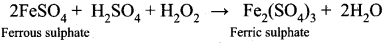• H2O2 act as reducing agent in basic medium. For example,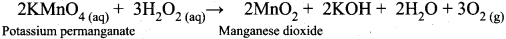>

Question 30.
Do you think that heavy water can be used for drinking purposes ?

• Heavy water (D2O) contains a proton and a neutron. This makes deuterium about twice as heavy as protium, but it is not radioactive. So heavy water is not radioactive.
• If you drink heavy water, you don’t need to worry about radiation poisoning. But it is not completely safe to drink, because the biochemical reaction in our cells are affected by the difference in the mass of hydrogen atoms.
• If you drink an appreciable volume of heavy water, you might feel dizzy because of the density difference. It would change the density of fluid in your inner ear. So it is unlikely to drink heavy water.

Question 31.
What is water-gas shift reaction?
The carbon monoxide of water gas can be converted to carbon dioxide by mixing the gas mixture with more steam at 400°C and passed over a shift converter containing iron/copper catalysts. This reaction is called water-gas shift reaction.
CO + H2O → CO+ H2

Question 32.
Justify the position of hydrogen in the periodic table?
Hydrogen resembles alkali metals in the following aspects.

1. Electronic configuration Is1 as alkali metals have ns1.
2. Hydrogen forms unipositive H+ ion like alkali metals Na+, K+.
3. Hydrogen form halides (HX), oxides (H2O) peroxide (H2O2) like alkali metals (NaX. Na2O, Na2O2).
4. Hydrogen also acts as reducing agent like alkali metals. Hydrogen resembles halogens in the following aspects.
5. Hydrogen has a tendency to gain one electron to form hydride ion (H) as halogens to form halide ion. (X).
6. Comparing the properties of hydrogen with alkali metals and with halogens, we can conclude that hydrogen resembles more alkali metals. In most of the compounds hydrogen exist in +1 oxidation state.
7. Therefore, it is reasonable to place the hydrogen in group 1 along with alkali metals as shown in the latest periodic table published by IUPAC.

Question 33.
What are isotopes? Write the names of isotopes of hydrogen.

1. Isotopes are atoms of the same element that have the same atomic number but having different mass numbers (or) Isotopes are atoms with the same number of protons and electrons but differ in number of neutrons.
2. Hydrogen has three naturally occuring isotopes namely Protium (1H1), Deuterium (1H2) and Tritium (1H3).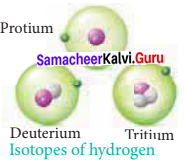Question 34.
Give the uses of heavy water.

1. Heavy water is used as moderator in nuclear reactors as it can lower the energies of fast moving neutrons.
2. D2O is commonly used as an tracer to study organic reaction mechanisms and mechanism of metabolic reactions.
3. It is also used as a coolant in nuclear reactors as it absorbs the heat generated.

Question 35.
Explain the exchange reactions of deuterium.
Deuterium can replace reversibly hydrogen in compounds either partially or completely depending upon the reaction conditions. These reactions occur in the presence of deuterium.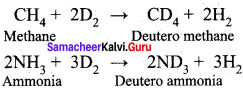Question 36.
How do you convert para hydrogen into ortho hydrogen ?
Para hydrogen can be converted into ortho hydrogen by the following ways:

• By treating with catalysts platinum or iron.
• By passing an electric discharge
• By heating > 800°C.
• By mixing with paramagnetic molecules such as O2, NO, NO2.
• By treating with nascent/atomic hydrogen.

Question 37.
Mention the uses of deuterium.

• Deuterium is used as a tracer element.
• Deuterium is used to study the movement of ground water by isotopic effect.

Question 38.
Explain preparation of hydrogen using electrolysis.
High purity of hydrogen (>99.9%) is obtained by the electrolysis of water containing traces of acid or alkali or electrolysis of aqueous solution of sodium hydroxide or potassium hydroxide using a nickel anode and iron cathode. This process is not economical for large scale production.
At anode : 2OH → H2O + ½ O2 + 2e
At cathode : 2H2O + 2e → 2OH + H2
Overall reaction : H2O → H2 + 14 O2

Question 39.
A groups metal (A) which is present in common salt reacts with (B) to give compound (C) in which hydrogen is present in -1 oxidation state. (B) on reaction with a gas (C) to give universal solvent (D). The compound (D) on reacts with (A) to give (B), a strong base. Identify A, B, C, D and E. Explain the reactions.
1.Group (1) metal (A) is present in common salt NaCl. So, (A) is sodium – Na.
2. Sodium reacts with hydrogen (B) to give sodium hydride – NaH (C) in which hydrogen is in -1 oxidation state.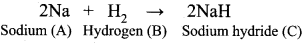3. Hydrogen on reaction with oxygen (O2) gas which is (C) to give a universal solvent water (D).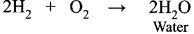4. Water (D) reacts with sodium metal (A) to give a strong base sodium hydroxide NaOH which is (E).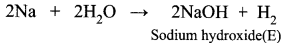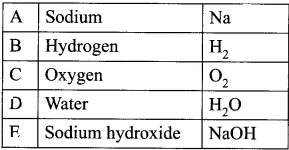Question 40.
An isotope of hydrogen (A) reacts with diatomic molecule of element which occupies group number 16 and period number 2 to give compound (B) is used as a modulator in nuclear reaction. (A) adds on to a compound (C), which has the molecular formula C3H6 to give (D). Identify A, B, C and D.
1. An isotope of hydrogen Deuterium (A) reacts with diatomic molecule of element belongs to group number 16 and period number 2 oxygen O2 to give a compound (B) which is heavy water D2O. D2O is used as a moderator in nuclear reaction.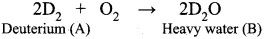2. Deuterium reacts with C3H6 propane (C) to give Deutero propane C2D6 (D).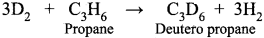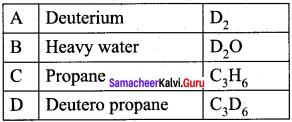Question 41.
NH3 has exceptionally high melting point and boiling point as compared to those of the hydrides of the remaining element of group 15- Explain.

1. NH3 has exceptionally high melting point and boiling point due to hydrogen bonding between NH3 molecules.
2. Each molecule can form a maximum of 4 hydrogen bonds but on average 1 hydrogen bond per molecule as there is only one lone pair on NH3 available for hydrogen bonding.
3. Hydrogen bonding is strong intermolecular attraction as H on NH3 acts like a proton due to partial positive on it whole N has the partial negative charge. Thus when the very polarized H comes close to a N atom in another NH3 molecule, a very strong hydrogen bond is formed.
4. Due to much strong intermolecular interactions compared to weaker permanent dipole-dipole interactions between other XH3 molecules in group 15, large amount of energy are required to overcome the forces, giving it the highest boiling point and highest melting point.

Question 42.
Why interstitial hydrides have a lower density than the parent metal.

• d block elements form metallic or interstitial hydrides, on heating with dihydrogen under pressure.
• Hydrogen atom being small in size occupy some in the metallic lattice producing distortion without any change in its type.
• The densities of these hydrides are lower than those of metals from which they are formed since the crystal lattice expands due to the inclusion of dihydrogen.

Question 43.
How do you expect the metallic hydrides to be useful for hydrogen storage?
In metallic hydrides, hydrogen is adsorbed as H-atoms. Due to the adsorption of H atoms the metal lattice expands and become unstable. Thus, when metallic hydride is heated, it decomposes to form hydrogen and finely divided metal. The hydrogen evolved can be used as fuel.

Question 44.
Arrange NH3, H2O and HF in the order of increasing magnitude of hydrogen bonding and explain the basis for your arrangement.

• Increasing magnitude of hydrogen bonding among NH3, H2O and HF is
HF>H2O>NH3
• The extent of hydrogen bonding depends upon electronegativity and the number of hydrogne atoms available for bonding.
• Among N, F nd O the increasing order of their electronegativities are
N<O H2O>NH3.

Question 45.
Compare the structures of H2O and H2O2.
In water, O is sp3 hybridized. Due to stronger lone pair-lone pair repulsions than bond pair-bond pair repulsions, the HOH bond angle decreases from 109.5° to 104.5°. Thus water molecule has a bent structure.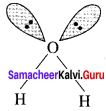H2O2 has a non-planar structure. The 0 – H bonds are in different planes. Thus, the structure of H2O2 is like an open book.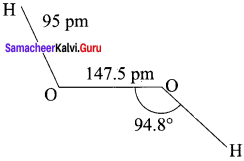### Samacheer Kalvi 11th Chemistry Hydrogen Additional Questions Solved

Question 1.
Which one of the following element mostly present in the sun and the stars?
(a) Hydrogen
(b) Lithium
(c) Helium
(d) Beryllium
(a) Hydrogen

Question 2.
is the most abundant 90% of all atoms…………
(a) Lithium
(b) Hydrogen
(c) Oxygen
(d) Silicon
(A) Hydrogen

Question 3.
At room temperature normal hydrogen consists of …………….
(a) 25% ortho form + 75% para form
(b) 50% ortho form + 50% para form
(c) 75% ortho form + 25% para form
(d) 60% ortho form + 40% para form
(c) 75% ortho form + 25% para form

Question 4.
Which one of the metal is used to convert para hydrogen into ortho hydrogen?
(a) Copper
(b) Aluminium
(c) Sodium
(d) Platinum
(d) Platinum

Question 5.
Consider the following statements…………….
(i) In ortho form of hydrogen molecule, the nuclear spins are opposed to each other
(ii) The magnetic moment of para hydrogen is twice that of ortho hydrogen
(iii) By passing an electric discharge, para hydrogen can be converted into ortho hydrogen. Which of the above statement is/are not correct?
(a) (i) only
(b) (iii) only
(c) (i) and (ii)
(d) (ii) and (iii)
(c) (i) and (ii)

Question 6.
Which of the following is not used in the conversion of para hydrogen into ortho hydrogen?
(a) by heating more than 800°C
(b) by passing an electric discharge
(c) by mixing with atomic hydrogen
(d) by mixing with diamagnetic molecules
(d) by mixing with diamagnetic molecules

Question 7.
The magnetic moment of para hydrogen is ……………
(a) one
(b) zero
(c) twice
(d) maximum
(b) zero

Question 8.
Which one of the following does not contain neutron?
(a) ordinary hydrogen
(b) Heavy hydrogen
(d) Deuterium
(a) ordinary hydrogen

Question 9.
The half life period of tritium is ……………..
(a) 123.3 year
(b) 12.33 years
(c) 1 year
(d) 1600 years
(a) 12.33 years

Question 10.
Which of the following is used in illumination of wrist watches?
(a) Phosphorous
(c) Tritium
(d) Deuterium
(c) Tritium

Question 11.
Which one of the following is used to study the movements of ground water?
(a) Deuterium
(b) Protium
(c) Tritium
(d) HD
(a) Deuterium

Question 12.
By which rays nuclear reactions are induced in upper atmosphere to produce tritium?
(a) α-rays
(b) β-rays
(c) γ-rays
(d) cosmic rays
(d) cosmic rays

Question 13.
Which of the following is produced by bombardment of neutrons with lithium?
(a) Deuterium
(b) Protium
(c) Tritium
(d) Beryllium
(c) Tritium

Question 14.
Consider the following statements ……………..
(i) Tritium is a beta emitting radioactive isotope of hydrogen.
(ii) Deuterium is known as heavy hydrogen.
(iii) Deuterium is used in emergency exit signs.
Which of the following statement is/are not correct?
(a) (i) only
(b) (iii) only
(c) (i) and (ii)
(d) (i) (ii) and (iii)
(b) (iii) only

Question 15.
Which of the following mixture of gases is called water gas?
(a) CO2(g)  + H2(g)
(b) CO2(g) + N2(g)
(c) CO(g) + H2(g)
(d) N2(g) + H2(g)
(c) CO(g) + H2(g)

Question 16.
Consider the following statements.
(i)Hydrogen is a colourless, odourless, tasteless heavy and highly inflammable gas.
(ii) Hydrogen is a good reducing agent.
(ii) Hydrogen can be liquefied under low pressure and high temperature.
Which of the above statements is/are not correct?
(a) (i) only
(b) (ii) only (c)
(c) (i) and (iii)
(d) (ii) and (Hi)
(c) (i) and (iii)

Question 17.
The products formed during the cracking long chain hydrocarbon C6H12 are
(a) CO2 + H2O
(b) CO + H2
(c) C6H10 + H2
(d) C6H6 + 3H2
(d) C6H6 + 3H2

Question 18.
Which one of the following is manufactured in Haber’s process?
(a) SO3
(b) NH3
(C) N2
(d) H2
(b) NH3

Question 19.
Hydrogen combines with carbon monoxide in the presence of copper catalyst will synthesise
(a) Ethanol
(b) Methane
(c) Methanol
(d) Methanal
(c) Methanol

Question 20.
Match the List-I and List-II using the correct code given below the list.
List-I
A. Hydrogenation of unsaturated vegetable oils
B. Calcium hydride
C. Liquid hydrogen
D. Atomic hydrogen

List-II
1. Rocket fuel
2. Welding of metals
3. Desiccant
4. Margarine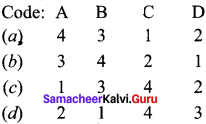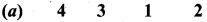Question 21.
Statement-I: Hydrogen is placed at the top of the group which is in-line with the latest periodic table.
Statement-II: Hydrogen has a tendency to lose its electron to form H+, thus showing electropositive character like alkali metals. On the other hand, hydrogen has a tendency to gain an electron to yield H , thus showing electronegative character like halogens.
(a) Statements-I and II are correct and Statement-II is the correct explanation of statement-I.
(b) Statements-I and II are correct but Statement-II is not the correct explanation of statement-I.
(c) Statement-I is correct but Statement-II is wrong.
(d) Statement-I is wrong but Statement-II is correct.
(a) Statements-I and II are correct and Statement-II is the correct explanation of statement-I.

Question 22.
Statement-I: The magnetic moment of para-hydrogen is zero.
Statement-II: The spins of two hydrogen atoms in para H2 molecule neutralise each other.
(a) Statements-I and II are correct and Statement-II is the correct explanation of statement-I.
(b) Statements-I and II are correct but Statement-II is not the correct explanation of statement-I.
(c) Statement-I is correct but Statement-II is wrong.
(d) Statement-I is wrong but Statement-II is correct.
(a) Statements-I and II are correct and Statement-II is the correct explanation of statement-I.

Question 23.
Which of the following process is important in food industry?
(a) Dehydration
(b) Dehalogenation
(c) Hydrogenation
(d) Carboxylation
(c) Hydrogenation

Question 24.
Which of the following is used as desiccants to remove moisture from organic solvents?
(a) Calcium hydride
(b) LiAlH4
(c) Sodium boro hydride
(d) Sodium hydride
(a) Calcium hydride

Question 25.
Which of the following is used for cutting and welding?
(a) Atomic hydrogen and oxy hydrogen torches
(b) Liquid hydrogen
(c) Calcium hydride
(d) Sodium boro hydride
(a) Atomic hydrogen and oxy hydrogen torches

Question 26.
Liquid hydrogen is used as
(a) a rocket fuel as well as in space research
(b) fuel cells for generating electrical energy
(c) cutting and welding torch
(d) desiccant to remove moisture from organic solvent
(a) a rocket fuel as well as in space research

Question 27.
Which one of the following is a universal solvent?
(a) Alcohol
(b) Ether
(c) CCl4
(d) Water
(d) Water

Question 28.
At the temperature conditions of the earth (300K) the OPR of H2O is ……………
(a) 2.5
(b) 3
(c) 1
(d) zero
(b) 3

Question 29.
Water does not react with
(a) Sodium
(b) Magnesium
(c) Beryllium
(d) Calcium
(c) Beryllium

Question 30.
Which of the following does not have any effect with water?
(a) Sodium
(b) Iron
(d) Mercury
(d) Mercury

Question 31.
Which set of the metals do not have any effect on water?
(a) Ag, Au, Pt
(b) Na, Mg, Al
(c) Fe, Ca, Zn
(d) Fe, Pb, Na
(a) Ag, Au, Pt

Question 32.
Which of the following non-metal reacts with ordinary water?
(a) Carbon
(b) Sulphur
(c) Chlorine
(d) Phosphorous
(c) Chlorine

Question 33.
Consider the following statements.
(i) Silver, Gold, Mercury and Platinum do not have any effect on water.
(ii) Carbon, Sulphur and Phosphorous do not react with water.
(iii) Beryllium reacts with water less violently.
Which of the following statements is/are not correct?
(a) (i) only
(b) (ii) and (iii)
(c) (iii) only
(d) (ii) only
(c) (iii) only

Question 34.
Which one of the following is used as a bleach?
(a) Cl2 water
(b) Br, water
(c) Water gas
(d) Liquid hydrogen
(a) Cl2 water

Question 35.
Water is an/a oxide.
(a) neutral
(b) acidic
(c) basic
(d) amphoteric
(d) amphoteric

Question 36.
Permanent hardness of water is removed by
(a) boiling
(b) lime
(c) washing soda
(d) chlorine
(c) washing soda

Question 37.
Which one of the following is used as water softener?
(a) Zeolites
(b) lime
(c) washing soda
(d) bleaching powder
(a) Zeolites

Question 38.
In chelating method of softening of hard water is used.
(a) magnesia
(b) lime
(c) EDTA
(d) washing soda
(c) EDTA

Question 39.
Which of the following is used to remove toxic and heavy metals from water?
(a) zeolites
(b) magnesia
(c) Bleaching agent
(d) lime
(a) zeolites

Question 40.
Match the List-I and List-Il using the code given below the list.
List-I
A. Heavy water
B. Hydrogen peroxide
C. Heavy hydrogen
D. Lithium Aluminium hydride

List-Il
1. Antiseptic
2. Moderator
3. Reducing agent
4. Tracer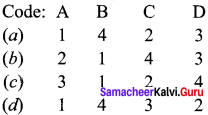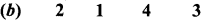Question 41.
Consider the following statements.
(i) H2O2 is a powerful oxidising agent.
(ii) H2O2 is stored in dark coloured bottles
(iii) H2O2 is used as moderator in nuclear reactors.
Which of the above statements is/are not correct.
(a) (i) only
(b) (i) and (ii)
(c) (iii) only
(d) (i), (ii) and (iii)
(c) (iii) only

Question 42.
Statement-I: Heavy water has been widely used as moderator in nuclear reactors.
Statement-Il: Heavy water can lower the energies of fast moving neutrons.
(a) Statements-I and II are correct and Statement-Il is the correct explanation of statement-I.
(b) Statements-I and II are correct but Statement-IT is not the correct explanation of statement-I;
(c) Statement-I is correct but Statement-Il is wrong.
(d) Statement-I is wrong but Statement-Il is correct.
(a) Statements-I and II are correct and Statement-Ills the correct explanation of statement-I.

Question 43.
Which one of the following is prepared in industry by the auto oxidation of 2-alkylanthraquional?
(a) Heavy water
(b) Deuterium
(c) Hydrogen peroxide
(d) Tritium
(c) Hydrogen peroxide

Question 44.
Which one of the following is an ionic or saline hydride?
(a) SiH4
(b) GeH4
(c) B2H6
(cl) LiH
(d) LiH

Question 45.
Which one of the following is an electron deficient hydride?
(a) C2H6
(b) B2H6
(c) GeH4
(d) CH4
(b) B2H6

Question 46.
Which of the following pair is an electron rich hydride?
(a) NH3, H2O
(b) CH4, C2H6
(c) B2H6, GeH4
(d) CH4, SiH4
(a) NH3, H2O

Question 47.
Which one of the following is not a covalent hydride?
(a) Ammonia
(b) Methane
(c) Lithium hydride
(d) water
(e) Lithium hydride

Question 48.
Metallic hydrides are otherwise called …………….
(a) Salt hydrides
(b) Saline hydrides
(c) molecular hydrides
(d) Interstitial hydrides
(d) Interstitial hydrides

Question 49.
Which one of the following is used for hydrogen storage applications?
(a) Saline hydrides
(b) Interstitial hydrides
(c) covalent hydrides
(d) molecular hydrides
(b) Interstitial hydrides

Question 50.
Which one of the following is known as Hydrogen sponge?
(a) Lithium hydride
(b) Diborane
(d) Ammonia

Question 51.
Which of the following is the correct order of stability of bonds?
(a) Hydrogen bond < CovaLent bond < Vanderwaals bond
(b) Vanderwaals bond < Hydrogen bond <Covalent, bond
(c) Vanderwaals bond > Hydrogen bond > Covalent bond
(d) Covalent bond < Hydrogen bond <Vanderwaals bond
(b) Vanderwaals bond < Hydrogen bond <Covalent, bond

Question 52.
Which one of the following does not have intramolecular hydrogen bonding?
(a) water
(b) o-nitrophenol
(c) Salicylaldehyde
(d) Salicylic acid
(a) water

Question 53.
Which of the following contains intramolecular hydrogen bonding?
(a) Acetic acid
(b) o-nitrophenol
(c) Hydrogen fluoride
(d) water
(b) o-nitrophenol

Question 54.
Consider the following statements.
(i) In ice, each oxygen atom is surrounded by hydrogen atoms tetrahedrally to four water molecules.
(ii) Acetic acid exists as dimer due to intra molecular hydrogen bonding.
(iii) Strong hydrogen bonds lead to an increase in the melting and boiling points.
Which of the above statements is/are not correct? ,
(a) (ii) only
(b) (i) and (iii)
(c) (i) (ii) and (iii)
(d) (i) only
(a) (ii) only

Question 55.
Which one of the following is an example for Clatharate hydrate?
(a) CuSO4.5H2O
(b) Na2CO3. 10H2O
(c) CH4. 20 H2O
(d) FeSO3.7H2O
(c) CH4. 20 H2O

Question 56.
Which one of the following is not a crystalline hydrate?
(a) CH4. 20H2O
(b) Na2,CO3. 10H2O
(c) CuSO4.5H2O
(d) FeSO4.7H20
(a) CH4. 20H2O

Question 57.
Statement-I: Hydrogen can be used as a clean burning fuel.
Statement-II: Hydrogen on combustion give only water as end product and it is free from pollutants.
(a) Statements-I and li are correct and Statement-lI is the correct explanation of statement-I.
(b) Statements-I and II are correct but Statement-Il is not the correct explanation of statement-I.
(e) Statement-I is correct but Statement-Il is wrong.
(d) Statement-I is wrong but Statement-lI is correct.
(a) Statements-I and II are correct and Statement-Il is the correct explanation of statement-I.

Question 58.
Which isotope of hydrogen is radioactive?
(a) Protium
(b) Deuterium
(c) Tritium
(d) H
(c) Tritium

Question 59.
Which type of elements form interstitial hydrides ………..
(a) s-block and p-block
(b) p-block only
(c) d-block and f-block
(d) s-block only
(c) d-block and f-block

Question 60.
Which of the following is named as perhydrol and used as an antiseptic?
(a) D2O
(b) H2O2
(c) NaH
(d) B2H6
(b) H2O2

Question 61.
Which of the following causes temporary hardness of water?
(a) MgCl2
(b) Na2SO4
(c) Mg(HCO3)2
(d) NaCl
(c) Mg(HCO3)2

Question 62.
Which of the following can oxidise Hydrogen peroxide?
(a) acidified KMnO4
(b) Cu
(c) dil. HNO4
(d) CrO2Cl2
(a) acidified KMnO4

Question 63.
Which type of hydrides are generally non-stoichiometric in nature?
(a) Metallic hydride
(b) Covalent hydrides
(c) Ionic hydride
(d) Saline hydride
(a) Metallic hydride

Question 64.
Hydrogen gas is generally prepared by the ………….
(a) reaction of granulated zinc with dilute H2SO4
(b) reaction of zinc with cone, H2SO4
(c) reaction of pure zinc with dil. H2SO4
(d) action of stream on red hot coke
(a) reaction of granulated zinc with dilute H2SO4

Question 65.
The higher density of water than that of ice is due to ……………
(a) dipole-dipole interaction
(b) dipole-induced dipole interaction
(c) hydrogen bonding
(d) all of these
(c) hydrogen bonding

Question 66.
Hydrogen resembles halogens in many respects for which several factors are responsible. Of the following factors which one is most important in this respect?
(a) Its tendency to lose an electron to form a cation.
(b) Its tendency to gain an electron to attain stable electronic configuration.
(c) Its low negative electron gain enthalpy value.
(d) Its small size.
(b) Its tendency to gain an electron to attain stable electronic configuration.

Question 67.
Metal hydrides are ionic, covalent or molecular in nature. Among L1H, NaH, KH, RbH, CsH, the correct order of increasing ionic character is ………….
(a) LiH>NaH>CsH>KH>RbH
(b) LiH<NaH<KH<RbHCsH>NaH>Kil>LIH
(d) NaH>CsH>RbH>LiH>KH
(b) LiH<NaH<KH<RbH<CsH

Question 68.
Statement-I: Permanent hardness of water is removed by treatment with washing soda ………..
Statement-II: Washing soda reacts with soluble magnesium and calcium sulphate to form insoluble carbonate.
(a) Statements-I and II are correct and Statement-Il is the correct explanation of statement-I.
(b) Statements-I and II are correct but Statement-Il is not the correct explanation of statement-I.
(c) Statement-I is correct but Statement-II is wrong.
(d) Statement-I is wrong but Statement-II is correct.
(a) Statements-I and II are correct and Statement-Ills the correct explanation of statement-I.

Question 69.
Which of the following is a saline hydride?
(a) HCl
(b) NH3
(c) NaH
(d) PbH
(e) NaH

Question 70.
Which metal does not liberate H2 gas from dilute aqueous hydrochloric acid at 298 K?
(a) Mg
(b) Zn
(c) Al
(d) Cu
(d) Cu

Question 71.
When zeolite is treated with hard water, the sodium ions are exchanged with …………
(a) H+ ions and Cl ions
(b) Ca2+ ions
(c) Cl ions
(d) Ca2+ ions and Mg2+ ions
(d) Ca2+ ions and Mg2+ ions

Question 72.
The most abundant element in the universe is …………..
(a) Carbon
(b) Nitrogen
(c) Silicon
(d) Hydrogen
(d) Hydrogen

Question 73.
Which of the following can effectively remove all types of hardness of water?
(a) Soap
(b) Slaked lime
(c) Washing soda
(d) Zeolite
(a) Soap

Question 74.
A commercial sample of H2O2 is labelled as 100 volume. Its percentage strength is nearly
(a) 10%
(b) 30%
(c) 100%
(d) 90%
(b) 30%

Question 75.
Which of the following will not produce di hydrogen gas?
(a) Cu + dil (HCl)
(b) CH2(g) + H2O(g)
(c) Zn + dil. HCl
(cl) C(s) + H2O(g)
(a) Cu + dil (HCl)

Samacheer Kalvi 11th Chemistry Hydrogen 2-Mark Questions
Question 1.
Draw and define ortho and para hydrogen molecule.
Molecular hydrogen have oriho and para form in which the nuclear spins are aligned or opposed, respectively.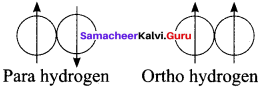Question 2.
What is the nuclear reaction that take place in the sun and other stars?
The sun and other stars are composed mainly of 85 – 95% hydrogen which generates their energy by nuclear fusion of hydrogen nuclei into helium.

Question 3.
Mention the uses of tritium.

• Tritium has replaced radium in application such as emergency exit sign.
• Tritium is used in illumination of wrist watches.

Question 4.
Draw the structures of three isotopes of hydrogen, Hydrogen Deuterium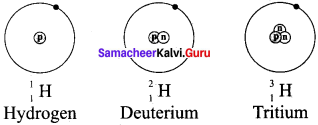Question 5.
What is the half life period of tritium? How is it undergoes radioactive disintegration?

• The half life of tritium = 12.33 years.
• Tritium is a beta-emitting radioactive isotope of hydrogen.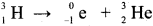Question 6.
Why hydrogen gas is used as fuel?
Hydrogen burns in air, virtually free from pollution and produces significant amount of energy. This reaction is used in fuel cells to generate electricity.
2H2(g) + O2(g) → 2H2O(l) + energy

Question 7.
How ammonia is manufactured from Hydrogen? Give the uses of ammonia.

• Ammonia is manufactured by Habe?s prôcess in which the largest consumer of hydrogen is used.
N2(g) + 3H2(g) ⇌ 2 NH3(g)
• Ammonia is employed for the manufacture of nitric acid, fertilizers and explosives.

Question 8.
how ¡s methanol synthesized from hydrogen? Give the uses of methanol.

• Huge quantities of hydrogen are used for the synthesis of methanol from carbon monoxide in presence of copper catalyst.
CO(g) + 2H2(g) → CH3OH(l)
•  Methanol is an industrial solvent and a starting material for the manufacture of formaldehyde used in makihg plastics.

Question 9.
What is hydrogenation? Give one example.
Hydrogenation is a reaction in which addition of hydrogen to an alkene /alkyne. Compounds containing multiple bonds.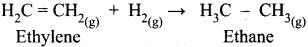Question 10.
How is hydrogen used in metallurgy? Prove it with an example.
In metallurgy, for the extraction of pure metals from their mineral sources, hydrogen is used to reduce many metal oxides to metals at high temperatures.
CuO(s) +H2(g) → Cu(s) +H(2)O(g)

Question 11.
How alkali metals react with water? Give an equation?
The most reactive alkali metals decompose water in the cold with the evolution of hydrogen and leaving an alkali solution.
2Na(s) +2H2O(l) → 2NaOH(aq) +H2(g)

Question 12.
What happens when steam is passed over red hot iron?
When steam is passed over red hot iron, iron oxide will be formed with the release of hydrogen.
3Fe(s) + 4H2O(l) → Fe3O + 4H4(g)

Question 13.
Explain the action of chlorine with water.
Chlorine reacts with the water to form hydrochloric acid and hypochiorous acid.
Cl2(g) + H2O(l) → HCl(aq) + HOCl(aq)

Question 14.
What is temporary hardness of water? How is it removed?
Temporary hardness of water is due to the presence of soluble bicarbonates of magnesium and calcium. By heating / boiling, these salts decomposed into insoluble carbonate and hydroxide, respectively. The resulting precipitates can be removed by filtration.
Ca (HCO3)2(aq) → CaCO3(s) + H2O(l) + CO2(l)
Mg(HCO3)2(aq) → Mg(OH)2(s) + CO2(g)

Question 15.
What ¡s permanent hardness of water? How ¡t will be removed?
Permanent hardness of water is due to the presence of soluble salts of magnesium and calcium in the form of chlorides and suiphates in water. It can be removed by adding washing soda which reacts with these metal chlorides and suiphates in hard water to form insoluble carbonates.
MCl2(aq) + Na2CO3(aq) → MCO3(s) + 2NaCl(aq)
MSO4(aq) + Ni2 CO3(aq) → MCO3(s) + Na2SO4(s)
Where M = Ca or Mg.

Question 16.
How would you prepare Hydrogen peroxide?
Hydrogen peroxide can be prepared by adding a metal peroxide to dilute acid.
BaO2(s)+ H2SO4(aq) → BaSO4(s) + H3O2(aq)

Question 17.
H2O2 is always stored in plastic bottles. Why?
The aqueous solution of hydrogen peroxide is spontaneously disproportionate to give oxygen. The reaction is slow but it is explosive when it is catalyzed by metal or alkali dissolved from glass. For this reason, its solution are stored in plastic bottles.
H2O2(aq) → H2O(l) + ½ O2(g)

Question 18.
Why H2O2 ¡s used as mild antiseptic?
The oxidising property of hydrogen peroxide and harmless nature of its products such as water and oxygen, leads to oxidation of pollutants in water and act as a mild antiseptic.

Question 19.
Why the bond angle in solid phase of H2O2 is reduced when compared to gas phase of H2O2?
Both the gas phase and solid phase, H2O2 adopt a skew configuration due to the repulsive interaction of the OH bonds with lone pairs of electrons on each oxygen atoms. Indeed, it is the smallest molecule known to show hindered rotation about a single bond. In the solid phase, the dihedral angle is sensitive and hydrogen bonding decreases from 111.50 in the gas phase to 90.2 in the solid phase.

Question 20.
What is meant by 100 – volume hydrogen peroxide?
A 30% solution is marketed as 100 – volume hydrogen peroxide indicating that at STP, 100 volumes of oxygen are liberated per millimeter of the solution.

Question 21.
Prove that Hydrogen peroxide is a vigorous oxidizing agent and the solution of H2O2 is slightly acidic.
H2 O2(aq) + 2 H2O(l) ⇌ H3 O+(aq) + HO+2(aq)

H3O+ Hydronium ion formation proves that solution of H2O2 is acidic. Because it donates H+ to H2O to form H3O+ ion.
H2O2 oxidizes Ferrous sulphate to Ferric sulphate in acidic medium.
2FeSO4(aq) + H2SO4(aq) + H4O2(aq) ⇌ Fe2(SO4)3(aq)( + 2H2O(l)

Question 22.
What is meant by binary hydride? Give example.
A binary hydride is a compound formed by hydrogen with other electropositive elements including metals and non-metals, e.g., LiH or MgH2.

Question 23.
What are ternary hydrides? Give example?
Ternary hydrides are compounds in which the molecule is constituted by hydrogen and two types of elements, e.g., LiBH4 or LiAlH4.

Question 24.
What arc the different types of hydrides?
The hydrides are classified as Ionic, Covalent and Metallic Hydrides.
Ionic hydride – LiH
Covalent hydride – CH4
Metallic hydride -TiH

Question 25.
Why metallic hydrides are called interstitial hydrides? Give one example.
Metallic hydrides are usually obtained by hydrogenation of metals and alloys in which hydrogen occupies the interstitial sites (voids). Hence, they are called interstitial hydrides. e.g., PdH.

Question 26.
What is hydrogen bonding?
When a hydrogen atom (H) is covalently bonded to a highly electronegative atom such as fluorine (F) or oxygen (O) or nitrogen (N), the bond is polarized in such a way that the hydrogen atom is able to form a weak bond between the hydrogen atom of a molecule and the electronegative atom of a second molecule. The bond thus formed is a hydrogen bond and it is denoted by dotted lines (……)

Question 27.
What are the types of hydrogen bonding? Give example.
There are two types of hydrogen bonding.

• Intramolecular hydrogen bonding: It can occur with a molecule. e.g., o – nitrophenol.
• Intermolecular hydrogen bonding: It is formed between two molécules of same type or different type. e.g., H2O.

Question 28.
Explain about the type of bonding present in hydrogen fluoride?
In hydrogen fluoride (HF), for example, one molecule is strongly attracted to the fluorine on its neighboring hydrogen. In both liquid and solid, hydrogen fluoride forms long hydrogen bonded zig-zag chains as a consequence of the orientation of the lone pairs on the fluorine atoms.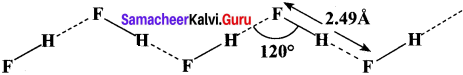Question 29.
Ice is less dense than water at 0°C. Justify this statement.
In ice, each oxygen atom is surrounded tetrahedrally by through hydrogen bonds to four water molecules. That is, the presence of two hydrogen atoms and two lone electron pairs (on oxygen) in each water molecule results in a three – dimensional structure. The arrangements creates an open structure, which in turn accounts for the fact that ice is less dense than water at 0°C.

Question 30.
Draw the structure of –

1. Acetic acid
2. Water.

1. Acetic acid: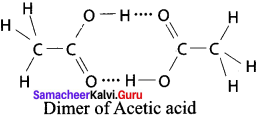2. Water: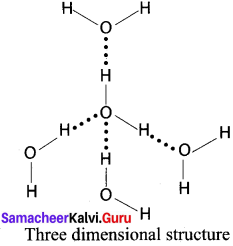Question 31.
Write a note about gas hydrates.
Gas hydrates are a kind of inclusion compounds, where gas molecules arc trapped in the crystal lattice having voids of right size, without being chemically bonded. An interesting hydrate is that of the hydronium ion (H2O) in the gas-phase, similar to methane hydrate. Each water molecule is bonded to three others in the dodecahedron.

Question 32.
Give the advantages of future fuel – Hydrogen.
Hydrogen is considered as a potential candidate for this purpose as it is a clean burning fuel. Hence, hydrogen can directly be used as a fuel and can replace existing gasoline (petrol) diesellkerosene powered engines, and indirectly be used with oxygen in fuel cells to generate electricity. One major advantage of using hydrogen is that the combustion product is essentially free from pollutants; the end product formed in both cases is water.

Question 33.
What do you understand by the term non-stoichiometric hydrides’? Do you expect this type of hydrides to be formed by alkali metals? Justify your answer.
Those hydrides which do not have fixed composition are called non-stoichiometric hydrides, and the composition varies with temperature and pressure. This type of hydrides are formed by d and f-block elements. They cannot be formed by alkali metals because alkali thetal hydrides form ionic hydrides.

Question 34.
How does the atomic hydrogen or oxy – hydrogen torch function for cutting and welding purposes? Explain.
When hydrogen is burnt in oxygen the reaction is highly exothermic, it produces very high temperature nearly 4000°C which is used for cutting and welding purposes.

Question 35.
How does H2O2 behave as bleaching agent?
Bleaching action of H2O2 is due to the oxidation of colouring matter by nascent oxygen.
H2 O2 → H2O(l) + O(g)

Question 36.
Can we use concentrated sulphuric acid and pure zinc in the preparation of dihydrogen?
Cone. H2SO4 cannot be used because it acts as an oxidizing agent also and gets reduced to SO2
Zn + 2H2SO4 (Conc.) → ZnSO4 + 2H2O + SO2
Pure Zn is not used because it is non-porous and reaction will be slow. The impurities in Zn help in constitute of electrochemical couple and speed up reaction.

Question 37.
Write the chemical reactions to show the amphoteric nature of water.
Water is amphotenc in nature and it behaves both as an acid as well as base. With acids stronger than itself (e.g., H2S) it behaves as a base and with bases stronger than itself (e.g., NH3) it acts as an acid.

1. As a base : H2O(l) + H2S(aq) → H3 O(aq) + HS(aq)
2. As an acid : H2O(l) + NH3(aq) → OH(aq) + NH+4(aq)

Question 38.
Why is hydrogen peroxide stored ¡n wax-lined plastic coloured bottles?
The decomposition of H2O2 occurs readily in the presence of rough surface (acting as catalyst). It is also decomposed by exposure of light. Therefore, wax-lined smooth surface and coloured bottles retard the decomposition of H2O2.

Samacheer Kalvi 11th Chemistry Hydrogen 3 – Mark Questions

Question 1.
Compare the properties of ortho and para hydrogen.

 s.no Properties Ortho hydrogen Para hydrogen 1. Melting point 13.95 K 13.83 K 2. Boiling point 20.39 K 20.26 K 3. Vapour pressurec Normal higher 4. Magnetic moment Twice zero

Question 2.
Compare the properties of isotopes of hydrogen.

 s.no Property Protium Deuterium Tritium 1. Atomic nature H D T 2. Atomic mass 1.008 2.014 3.016 3. Nuclear stability Stable Stable Radioactive 4. Molecular hydrogen H2 D2 T2 5. Abundance(%) 99.985 0.015 ᷉10-16 6. Molecular mass 2.016 4.028 6.032

Question 3.
Draw the structure of the isotopes of hydrogen and distinguish them.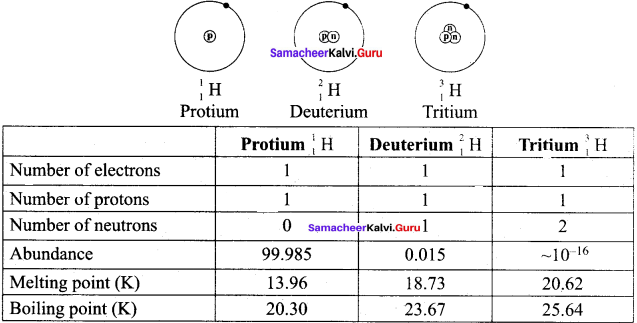Question 4.
Explain the different methods of preparation of Tritium with equation.
It occurs naturally as a result of nuclear reactions induced by cosmic rays in the upper atmosphere.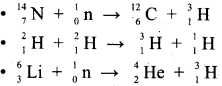Question 5.
How would you prepare hydrogen in the laboratory?
Small amounts of hydrogen are conveniently prepared in laboratory by the reaction of metals, such as zinc, iron and tin, with dilute acid.
Zn(s)  + 2HCl(aq) → ZnCl2(s) + H2(g)
In principle, any metal with a negative standard reduction potential will react with an acid to generate hydrogen.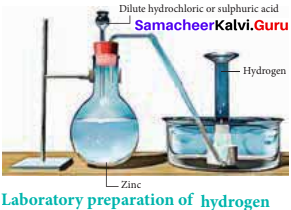Question 6.
What happens when hydrogen reacts with –

1. O2
2. Cl2
3. Na ?

1. 2 H2(g) + O2(g) → 2 H2O(l) – Water
2. H2(g) + Cl2(g) → 2 HCl(g) – Hydrogen Chloride
3. 2 Na(s) + H2(g) → 2 NaH(s) – Sodium hydride

Question 7.
Write a note about ortho water and para water.

1. Water exists in space in the interstellar clouds, ¡n proto-planetary disks, in the comets and icy satellites of the solar system, and on the Earth.
2. In particular, the ortho-to-para ratio (OPR) of water in space has recently received attention. Like hydrogen, water can also be classified into ortho-H2O and para-H2O, in which the directions are antiparallel.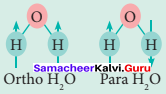3. At the temperature conditions of the Earth (300 K), the OPR of H2O is 3.
4. At low temperatures below (< 50 K) the amount of para-H2O increases. It is known that the OPR of water in interstellar clouds and comets has more para-H2O (OPR = 2.5) than on Earth.

Question 8.
Water ¡s an amphoteric oxide. Justify this statement.

1. Water is an amphoteric oxide. It has the ability to act an acid as well as a base. That is, water shows this behavior when it reacts with hydrogen chloride and ammonia.
2. When water reacts with ammonia, it behaves as an acid.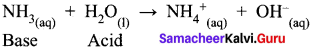3. When water reacts with hydrogen chloride, behaves as a base.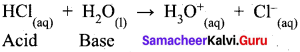So water is an amphoteric oxide.

Question 9.
Distinguish between Hard water and Soft water.
Hard water:

• Presence of magnesium and calcium in the form of bicarbonate, chloride and sulphate in water makes hard water.
• Cleaning capacity of soap is rcduced when used in hard water.
• When hard water is boiled deposits of insoluble carbonates of magnesium and calcium are obtained.

Soft water:

• Presence of soluble salts of calcium and magnesium in water makes it soft water.
• Cleaning capacity of soap is more when used in soft water.
• When soft water is boiled, there is no deposition of salts.

Question 10.
Explain the action of soap with hard water.

• The cleaning capacity of soap is reduced when used in hard water.
• Soaps are sodium or potassium salts of long chain fatty acids.
• When soap is added to hard water, the divalent magnesium and calcium cations present in hard water react with soap.
• The sodium salts present in soaps are converted to their corresponding magnesium and calcium salts which are precipitated as scum or precipitate.
M2+ + 2RCOONa (RCOO)2M(s) + 2Na+(aq);
Where, M Ca or Mg;
R = C17H35.

Question 11.
Describe about ion exchange method of softening water (or) Explain Zeolite (or) Permutit process.

1. Hardness can be removed by passing through an ion-exchange bed like zeohtes or resin containing column. Thus, the zeolites work as water softener.
2. Zeolites are hydrated sodium alumini-silicates with a general formula, NaO.Al2O3.xSiO2.yH2O (x = 2 – 10, y = 2 – 6). They have high ion exchange capacity.
3. The complex structure can represented as Na– Z with sodium as exchangeable cations. This method is called zeolite or permutit process.
4. Zeolites have porous structure in which the monovalent sodium ions are loosely held and can be exchanged with hardness producing divalent metal ions (Ca or Mg) in water.
Na2 – Z(s) + M2+(aq) → M-Z(s) + 2Na+(aq)
5. When exhausted, the materials can be regenerated by treating with aqueous sodium chloride. Hard minerals caught in the zeolite are released and they get replenished with sodium ions.
M-Z(s) + 2NaCl(aq) → Na(s)-Z(s) + MCl2(aq)

Question 12.
Explain about the exchange reactions of deuterium oxide.
When compounds containing hydrogen are treated with D2O, hydrogen undergoes an exchange for Deuterium.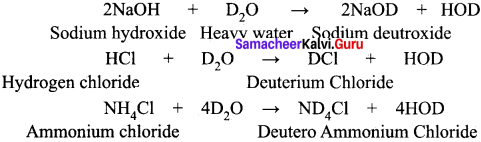Question 13.
Complete the following reactions.
A14C3 + D2O → ?
CaC2 + D2O →?
Mg3N2 + D2O →?
Ca3P2 + D2O →?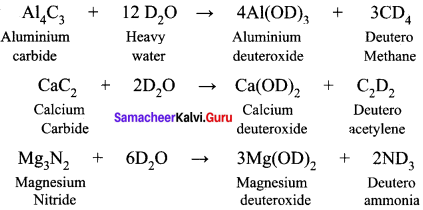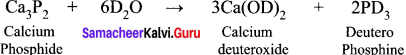Question 14.
What are the uses of hydrogen peroxide?

• H2O2 is used in water treatment to oxidize pollutants.
• H2O2 is used as a mild antiseptic.
• H2O2 is used as a bleach in textile, paper and hair-care industry.

Question 15.
Prove that H2O2 act as reducing agent ¡n alkaline medium.
In alkaline conditions, H2O2 act as a reducing agent.
2KMnO4(aq)(Potassium permanganate) + 3 H2SO4(aq) + 5H2O2(aq) → K2 SO4 + 2MnSO4 + 8H2 O(l) + 5O2(g)

Question 16.
Write a note about saline (or) ionic hydride.
Ionic hydrides composed of an electropositive metal, generally, an alkali or alkaline-earth metal (except beryllium and magnesium) formed by transferring of electrons from metal to hydrogen atoms. They can be prepared by the reaction of elements at about 400°C. These are salt-like, high-melting, white, crystalline solids having hydride ions (H) and metal cations (Mn+).
2Li(s) + H2(g) → 2LiH(s) (Lithiuinhydride)
2 Ca(s) + H2(g) → 2 CaH2(s) (Calcium hydride)

Question 17.
What are metallic hydrides? Explain about it.

• Metallic hydrides are obtained by hydrogenation of metals and alloys in which hydrogen occupies the interstitial sites (voids). Hence, they are called interstitial hydrides.
• The hydrides show properties similar to parent metals and hence they are also known as metallic hydrides.
• They arc mostly non-stoichiometric with variable composition (TiH1.5-1.818 and PdH0.6-0.8).
• Some are relatively light, inexpensive and thermally unstable which makes them useful for hydrogen storage applications. Example, TiH2, ZrH2, ZnH2.

Question 18.
What are intra molecular hydrogen bonding? Explain with an example.

1. Intramolecular hydrogen bonds are those which occur within one single molecule. This occurs between two functional groups within a molecule.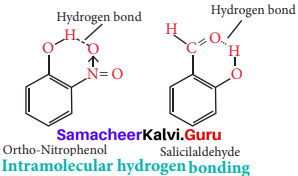2. An intramolecular hydrogen bond (dashed lines) joins the OH group to the doubly bonded oxygen atom of the carboxyl group on the same molecule. e.g., Salicylic acid.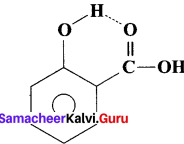3. Salicylic acid act as an analgesic and antipyretic.

Question 19.
What are intermolecular hydrogen bonds? Explain with example.

1. Intermolecular hydrogen bonds occur between two separate molecules. They can occur between any numbers of like or unlike molecules as long as hydrogen donors and acceptors are present in positions in which they can interact.
2. For e.g., Intermolecular hydrogen bonds can occur between ammonia molecules alone, between water molecules alone or between ammonia and water.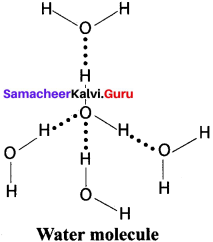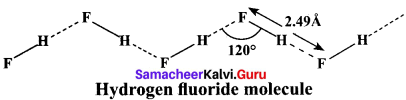Question 20.
Explain about the importance of hydrogen bonding ¡n proteins.

• Hydrogen bonds occur in complex biomolecules such as proteins and in biological systems.
• For example, hydrogen bonds play an important role in the structure of deoxyribonucleic acid (DNA), since it holds together the two helical nucleic acid chains.
• In these systems, hydrogen bonds are formed between specific pairs, for example. with a thymine unit in one chain bonding to an adenine unit in another; similarly, a cytosine unit in one chain bonds to a guanine unit in another.
• Intramolecular hydrogen bonding also plays an important role in the structure of polymers, both synthetic and natural.

Question 21.
What are Clatharate hydrate? Explain it with suitable example.

• Gas hydrates ¡n which the guest molecules are not bonded chemically but retained by the structure of host is called Clatharates.
• Water forms clatharate hydrates, e.g., methane hydrate (CH20H2O) which arc a type of ice that will bum when a lit match is held to it.
• The structure of methane hydrate is made of linked polyhedra that contains 20 hydrogen bonded water molecules forming a cage in which methane molecule is trapped.
• Deposits of methane clatharates occur naturally in deep sea bed.
• Hydrates are commonly obtained when water is frozen in presence of a gas such as argon, methane, etc.
• Most gases form hydrates under high pressure.

Question 22.
What are crystalline hydrates? Explain it with example.

1. In these, hydrogen bonding is very important. Often the water molecules serve to fill in the interstices and bind together structure.
2. A specific example is CuSO4  5H2O.
3. Although there are five water molecules for every divalent copper ion, only four are coordinated to the cation, it’s six-coordination being completed from sulphate anions. The fifth water molecule is held in place of hydrogen bonds, O – H – O, between it and two coordinated water molecules and then coordinate sulphate anion.
4. Water forms hydrated salts during crystallization. Examples, Na2 CO3 . 10H2O, FeSO4 .7H2O.
5. The water present in the hydrates is called as water of hydration.

Question 23.
What do you understand by –

1. Electron-deficient
2. Electron-precise
3. Electron-rich compounds of hydrogen? Provide justification with suitable examples.

1. Electron deficient hydrides:
Compounds in which central atom has incomplete octet, are called electron deficient hydrides. For example, BeH2 , BH3  are electron deficient hydrides
2. Electron precise hydrides:
Those compounds in which exact number of electrons are present in central atom or the central atom contains complete octet are called precise hydrides e.g., CH4 , SiH4, GeH4 etc. are precise hydrides.
3. Electron rich hydrides:
Those compounds in which central atom has one or more lone pair of excess electrons are called electron rich hydrides. e.g., NH3, H2O.

Question 24.
What causes the temporary and permanent hardness of water?
Temporary hardness of water is due to the presence of bicarbonates of calcium and magnesium in water i.e., Ca(HCO3)2 and Mg(HCO3) in water. Permanent hardness of water is due to the presence of soluble chlorides and suiphates of calcium and magnesium i.e., CaCl2, CaSO4, MgCl2 and MgSO4.

Question 25.
Write chemical reaction to show the amphoteric nature of water.
Water is amphoteric in nature because it acts as an acid.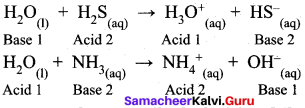Question 26.
What is the difference between the terms ‘hydrolysis’ and ‘hydration’?
Hydrolysis is a chemical reaction in which a substance reacts with water under neutral, acidic or alkaline conditions.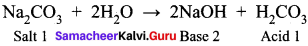Hydration on the other hand is the property of a chemical compound to take up molecules of water of crystallization and get hydrated.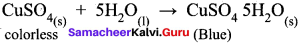Samacheer Kalvi 11th Chemistry Hydrogen 5-Mark Questions

Question 1.
Explain about the different industrial preparation of hydrogen.
In the large scale, hydrogen is produced currently by steam reforming of hydrocarbons. Steam and methane reacts with each other in the presence of nickel catalyst at 35 atm and at a temperature of 800°C gives hydrogen.
CH4(g) + H2O(g) → CO(g) +3H2(g)
Steam is passed over a red hot coke to produce carbon monoxide and hydrogen.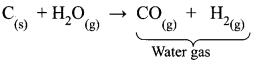Water is reduced to hydrogen with carbon monoxide by passing over iron oxide catalyst at 400°C.
CO(g) + H2O(g) → CO2(g) + H2(g)
Hydrogen is produced as a by-product in oil refining industry during the cracking of long chain hydrocarbons.
C6H12(g) → CH66(g) + 3H2(g)
Hydrogen is also obtained in the manufacture of chlonne and sodium hydroxide via electrolysis of a concentrated solution of sodium chloride.

Question 2.
Explain about the uses of hydrogen compounds.

• The hydrogen compounds such as sodium borohydride (NaBH4) and lithium aluminium hydride (LiAlH4) are commonly used as reducing agents in organic chemistry.
• Hydrides such as sodium hydride (NaH) and potassium hydride (KH) are used as strong bases in organic synthesis.
• Calcium hydride is used as desiccant to remove moisture from organic solvents.
• Hydride complexes are catalysts.
• Atomic hydrogen and oxy-hydrogen torches for cutting and welding.
• Hydrogen is used in fuel cells for generating electrical energy.
• Liquid hydrogen is used as a rocket fuel as well as in space research.
• Metallic hydrides are used in battery applications.

Question 3.
Describe the process of water softening and purification.

1. An idealised image of water softening process involves replacement of cations such as Mg, Ca and Fe in water with sodium ions.by a cation exchange zeolite. The ion exchange zeolites or resins are used to replace the Mg and Ca ions found in hard water with sodium ions.
2. They can be recharged by washing it with a solution containing a high concentration of sodium ions.
3. The calcium and magnesium ions migrate from the zeolite or resin being replaced by sodium ions from the solution until a new equilibrium is reached. That is, the salt is used to recharge an ion exchange medium, which itself is used to soften the water.
4. A couple of other methods, namely chelating method and reverse osmosis are also used to soften hard water. Chelating method employs a polydentate ligand such as EDTA, while reserve osmosis uses high pressure to force the water through a semi-permeable membrane.
5. In the case of water purification application, ion exchange zeolites or resins are used to remove toxic (eg., copper) and heavy metal (e.g., cadmium or lead) ions from solution, replacing them with harmless sodium or potassium ions.

Question 4.
(a) How is H2O2 prepared?
(b) Explain about the structure of H2O2.
(a) Hydrogen peroxide can be made by adding a metal peroxide to dilute acid.
BaO2(s) + H(s)SO4 → BaSO4(s) + H2O2(aq)
(b) Structure of H2O2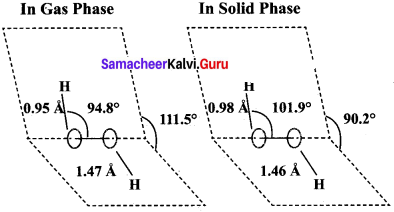1. H2O2 has a non-polar structure. The molecular dimensions in the gas phase and solid phase differ as shown in the figure.

2. Both in gas phase and solid phase, the H2O2 molecule adopt a skew configuration due to repulsive interaction of the – OH bonds with lone pairs of electrons on each oxygen atom.

3. Indeed, it is the smallest molecule known to show hindrance rotation about a single bond. in solid phase, the dihedral angle is sensitive and hydrogen bonding decreasing from 111.50 in the gas phase to 90.2°, in the solid phase.

4. Structurally, H2O2 is represented by the dihydroxyl formula in which the two O-H groups do not lie in the same plane. In the solid phase of molecule, the dihedral angle reduces to 90.2° due to hydrogen bonding and the O-O-H angle expands from 94.8° to 101.9°.

5. One way of explaining the shape of hydrogen peroxide is that the hydrogen atoms would lie on the pages of a partly opened book, and the oxygen atoms along the spin.

Question 5.
1. Hydrogen sponge (or) Metal hydride e.g., palladium-hydrogen system is a binary hydride (PdH).

2. Upon heating, H atoms diffuse through the metal to the surface and recombine to form molecular hydrogen. Since no other gas behaves this way with palladium, this process has been used to separate hydrogen gas from other gases:
2Pd(s) + H2(g) ⇌ 2PdH(s)

3. The hydrogen molecule readily adsorb on the palladium surface, where it dissociates into atomic hydrogen. The dissociated atoms dissolve into the interstices or voids (octahedral or tetrahedral) of the crystal lattice.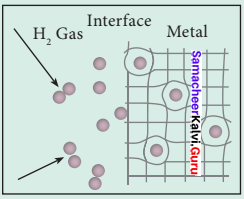4. Technically, the formation of metal hydride is by chemical reaction but it behaves like a physical storage method, i.e., it is absorbed and released like a water sponge. Such a reversible uptake of hydrogen in metals and alloys is also attractive for hydrogen Storage and for rechargeable metal hydride battery applications.

Question 6.
How are reducing agents in synthetic organic chemistry prepared?
Hydrogen has a tendency to react with reactive metals like lithium, sodium to give corresponding hydrides.
2Li+H2 → 2LiH
2Na+H2 → 2NaH
These hydrides are used as reducing agents in synthetic organic chemistry. It is also used to prepare important hydrides such as lithium aluminium hydride and sodium boro hydride (organic reducing agents).
4 LiH + AlCl3 → Li[AIH4] + 3 LiCl
4 NaH + B(OCH3)3 → Na[BH4] + 3 CH3ONa

Question 7.
How does water react with –
1. SiCl4
2. P4O10
1. Water reacts with SiCl4 to give silica.
SiCl4 + 4H2O → Si(OH)4 + 4HCl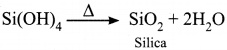2. Water reacts with P4O10 to give ortho phosphoric acid.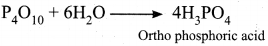Question 8.
Explain about the structure of CuSO4.5H2O
Copper sulphate pentahydrate CuSO4.5H2O. In this compound, 4 water molecules form coordinate bonds while the fifth water molecule present outside the coordination can form intermolecular hydrogen bond with another molecule as [Cu(H2O)2] SO2.H2O.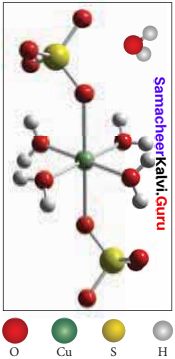Question 9.
How is hydrogen peroxide prepared on industrial scale?
On an industrial scale, hydrogen peroxide is prepared by auto oxidation of of 2-alkyl antliraquinol.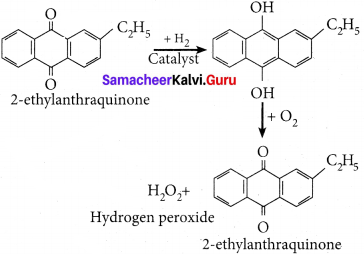Question 10.
How is hydrogen peroxide is used to restore the white colour of old paintings.
Hydrogen peroxide is used to restore the white colour which was lost due to the reaction of hydrogen suiphide in air with the white pigment Pb3(OH)2(CO3)2 to form black colored lead suiphide (PbS) Hydrogen peroxide oxidises black coloured lead suiphide to white coloured lead sulphate, there by restoring the colour.
PbS + 4H2O2 → PbSO2 + 4H2O

## How to Prepare using Samacheer Kalvi 11th Chemistry Chapter 4 Hydrogen Notes PDF?

Students must prepare for the upcoming exams from Samacheer Kalvi 11th Chemistry Chapter 4 Hydrogen Notes PDF by following certain essential steps which are provided below.

• Use Samacheer Kalvi 11th Chemistry Chapter 4 Hydrogen notes by paying attention to facts and ideas.
• Pay attention to the important topics
• Refer TN Board books as well as the books recommended.
• Correctly follow the notes to reduce the number of questions being answered in the exam incorrectly
• Highlight and explain the concepts in details.

## Frequently Asked Questions on Samacheer Kalvi 11th Chemistry Chapter 4 Hydrogen Notes

#### How to use Samacheer Kalvi 11th Chemistry Chapter 4 Hydrogen Notes for preparation??

Read TN Board thoroughly, make separate notes for points you forget, formulae, reactions, diagrams. Highlight important points in the book itself and make use of the space provided in the margin to jot down other important points on the same topic from different sources.

#### How to make notes for Samacheer Kalvi 11th Chemistry Chapter 4 Hydrogen exam?

Read from hand-made notes prepared after understanding concepts, refrain from replicating from the textbook. Use highlighters for important points. Revise from these notes regularly and formulate your own tricks, shortcuts and mnemonics, mappings etc.
Share: